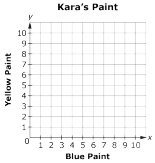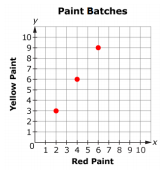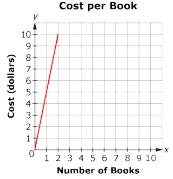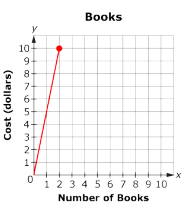# MAFS.7.RP.1.2Archived StandardExport Print
Recognize and represent proportional relationships between quantities.
1. Decide whether two quantities are in a proportional relationship, e.g., by testing for equivalent ratios in a table or graphing on a coordinate plane and observing whether the graph is a straight line through the origin.
2. Identify the constant of proportionality (unit rate) in tables, graphs, equations, diagrams, and verbal descriptions of proportional relationships.
3. Represent proportional relationships by equations. For example, if total cost t is proportional to the number n of items purchased at a constant price p, the relationship between the total cost and the number of items can be expressed as t = pn.
4. Explain what a point (x, y) on the graph of a proportional relationship means in terms of the situation, with special attention to the points (0, 0) and (1, r) where r is the unit rate.

### Remarks

Examples of Opportunities for In-Depth Focus

Students in grade 7 grow in their ability to recognize, represent, and analyze proportional relationships in various ways, including by using tables, graphs, and equations.
General Information
Subject Area: Mathematics
Domain-Subdomain: Ratios & Proportional Relationships
Cluster: Level 2: Basic Application of Skills & Concepts
Cluster: Analyze proportional relationships and use them to solve real-world and mathematical problems. (Major Cluster) -

Clusters should not be sorted from Major to Supporting and then taught in that order. To do so would strip the coherence of the mathematical ideas and miss the opportunity to enhance the major work of the grade with the supporting clusters.

Date of Last Rating: 02/14
Status: State Board Approved - Archived
Assessed: Yes
Test Item Specifications

• Assessment Limits :
Ratios should be expressed as fractions, with “:” or with words. Units may be the same or different across the two quantities.
• Calculator :

Neutral

• Context :

Allowable

Sample Test Items (5)

• Test Item #: Sample Item 2
• Question: Kara is mixing paint. Each batch has twice as much blue paint as yellow paint.

Plot points to represent the amount of blue and yellow paint used in three different-sized batches.• Difficulty: N/A
• Type: GRID: Graphic Response Item Display

• Test Item #: Sample Item 3
• Question: The points on the coordinate plane show the amount of red and yellow paint in each batch.Write an equation to represent the relationship between red paint, r, and yellow paint, y, in each batch.

• Difficulty: N/A
• Type: EE: Equation Editor

• Test Item #: Sample Item 4
• Question:

The graph below represents the rate for the cost of b books.Write an equation to represent the cost, c.

• Difficulty: N/A
• Type: EE: Equation Editor

• Test Item #: Sample Item 5
• Question: The ordered pair (1, 5) indicates the unit rate of books to cost on the graph shown.What does the point on the graph represent?

• Difficulty: N/A
• Type: OR: Open Response

## Related Courses

This benchmark is part of these courses.
1205020: M/J Accelerated Mathematics Grade 6 (Specifically in versions: 2014 - 2015, 2015 - 2020, 2020 - 2022, 2022 and beyond (current))
1205040: M/J Grade 7 Mathematics (Specifically in versions: 2014 - 2015, 2015 - 2022, 2022 and beyond (current))
1204000: M/J Foundational Skills in Mathematics 6-8 (Specifically in versions: 2014 - 2015, 2015 - 2022, 2022 and beyond (current))
7812020: Access M/J Grade 7 Mathematics (Specifically in versions: 2014 - 2015, 2015 - 2018, 2018 - 2019, 2019 - 2022, 2022 and beyond (current))
7912115: Fundamental Explorations in Mathematics 2 (Specifically in versions: 2013 - 2015, 2015 - 2017 (course terminated))

## Related Access Points

Alternate version of this benchmark for students with significant cognitive disabilities.

## Related Resources

Vetted resources educators can use to teach the concepts and skills in this benchmark.

## Educational Software / Tool

Free Graph Paper:

A variety of graph paper types for printing, including Cartesian, polar, engineering, isometric, logarithmic, hexagonal, probability, and Smith chart.

Type: Educational Software / Tool

## Formative Assessments

Identifying Constant of Proportionality in Equations:

Students are asked to identify and explain the constant of proportionality in three different equations.

Type: Formative Assessment

Teacher to Student Ratios:

Students are asked to graph four ordered pairs given in context and decide if the variables they represent are proportionally related.

Type: Formative Assessment

Constant of Proportionality Trip:

Students are asked to identify and explain the constant of proportionality given a verbal description and a diagram representing a proportional relationship.

Type: Formative Assessment

Writing An Equation:

Students are asked to write an equation to represent a proportional relationship depicted in a graph.

Type: Formative Assessment

Graphs of Proportional Relationships:

Students are asked to identify the graph of a proportional relationship.

Type: Formative Assessment

Babysitting Graph:

Students are given a graph that models the hourly earnings of a babysitter and are asked to interpret ordered pairs in context.

Type: Formative Assessment

Finding Constant of Proportionality:

Students are asked to determine the constant of proportionality using a table and a graph.

Type: Formative Assessment

Deciding If Proportional:

Students decide if two variables are proportionally related based on data given in a table.

Type: Formative Assessment

Serving Size:

Students are given the number of calories in a serving of oatmeal and are asked to write an equation that models the relationship between the size of the serving and the number of calories.

Type: Formative Assessment

## Lesson Plans

Guiding Grids: Math inspired self-portraits:

In this lesson students will create a proportional self portrait from a photo using a gridded drawing method and understand how a grid system can help accurately enlarge an image in a work of art.

Students will use mathematical concepts of scale, proportion and ratio, to complete their artwork and learn about the work of artist, Chuck Close.

Type: Lesson Plan

Read about a recent uncovering of mammoths to engage students in a discussion of radioactive dating. This is the first lesson in a unit of 4 lessons that integrates science, math, and computer science standards to teach the concept of half-lives and radioactive dating.

Type: Lesson Plan

Irrigation Station:

This STEM lesson, complete with a design challenge, helps students design, build, and test irrigation methods. Students will incorporate and develop math skills through solving proportions as they work in teams to solve an engineering challenge.

Type: Lesson Plan

How Fast Can One Travel on a Bicycle?:

Students investigate how the pedal and rear wheel gears affect the speed of a bicycle. A GeoGebra sketch is included that allows a simulation of the turning of the pedal and the rear wheel. A key goal is to provide an experience for the students to apply and integrate the key concepts in seventh grade mathematics in a familiar context.

Type: Lesson Plan

Cricket Songs:

Using a guided-inquiry model, students in a math or science class will use an experiment testing the effect of temperature on cricket chirping frequency to teach the concepts of representative vs random sampling, identifying directly proportional relationships, and highlight the differences between scientific theory and scientific law.

Type: Lesson Plan

Are Corresponding Leaf Veins Proportional to Leaf Height?:

Students will measure the length of different sized leaves and corresponding veins to determine proportionality.  Students will graph their results on a coordinate grid and write about their results.

Type: Lesson Plan

How Does It Compare?:

This lesson addresses part a. of the Standard. It introduces proportions and addresses solving proportion problems with ratio tables. Students will be able to identify whether a statement shows proportionality or is simply two non-proportional ratios. The focus will be on recognizing visual proportions as being equivalent values and will lead into recognizing ratios represented as fractions as equivalent or non-equivalent.

Type: Lesson Plan

Developing a Sense of Scale:

This lesson unit is intended to help you assess whether students recognize relationships of direct proportion and how well they solve problems that involve proportional reasoning. In particular, it is intended to help you identify those students who use inappropriate additive strategies in scaling problems, which have a multiplicative structure, rely on piecemeal and inefficient strategies such as doubling, halving, and decomposition, and have not developed a single multiplier strategy for solving proportionality problems and see multiplication as making numbers bigger, and division as making numbers smaller.

Type: Lesson Plan

Distinguishing Between Proportion and Non-proportion Situations:

This lesson is from the Mathematics Assessment Resource Service (MARS) collection of the Mathematics Assessment Project's (MAP) Classroom Challenges, and involves a review task, lesson task, and assessment task along with a PowerPoint.

In this lesson, students identify when two quantities vary in direct proportion to each other, distinguish between direct proportion and other functional relationships, and solve proportionality problems using efficient methods.

Type: Lesson Plan

Estimating: Counting Trees:

This lesson unit is intended to help you assess how well students are able to solve simple problems involving ratio and direct proportion, choose an appropriate sampling method, collect discrete data, and record their data using a frequency table.

Type: Lesson Plan

Back to the Past with the Geologic Time Scale:

This lesson introduces the geologic time scale and the concept of time segments being divided by major events in Earth's history. It gives students an opportunity to place various fossils into appropriate periods, observe the change in the complexity of fossils and draw conclusions regarding the change. Students complete a brace map including the eras and periods showing their understanding of parts to the whole within the geologic time scale. On day 2, students research an organism of their choice and trace it back to their most basic relative. Students then create a final product, such as a brochure, timeline or a poster, demonstrating the change of the organism over time. Students will be provided with a rubric that will guide them while they work on the final product.

Type: Lesson Plan

Recognizing Proportional Relationships to Develop Sense of Scale:

This 90-minute lesson (15-minute pre-lesson, 60-minute lesson and 15-minute follow up lesson or homework) asks students to analyze proportional relationships to solve real world and mathematical problems. The examples use recipes, paint, and buildings. Students begin by working individually, then in pairs or threes, and then as a whole class. Student will need calculators, large sheets of paper to make a poster and the lesson materials.

Type: Lesson Plan

Making a Scale Drawing:

Objective: Students will create a detailed scale drawing. Context: Students have used tools to measure length, solve proportions, and interpret scale drawings. They will continue to use ratio and proportion in the study of similar figures, percent, and probability.

Type: Lesson Plan

Increasing and Decreasing Quantities by a Percent:

This lesson unit is intended to help you assess how well students are able to interpret percent increase and decrease, and in particular, to identify and help students who have the following difficulties:

• Translating between percents, decimals, and fractions.
• Representing percent increase and decrease as multiplication.
• Recognizing the relationship between increases and decreases.

Type: Lesson Plan

Scientific calculations from a distant planet:

Students will act as mathematicians and scientists as they use models, observations and space science concepts to perform calculations and draw inferences regarding a fictional solar system with three planets in circular orbits around a sun. Among the calculations are estimates of the size of the home planet (using a method more than 2000 years old) and the relative distances of the planets from their sun.

Type: Lesson Plan

"Are My Values in Direct Variation?":

Students will learn that a direct variation is a proportional relationship that can be represented by a table, a graph, or an equation. They will also be able to recognize if they are dealing with a direct variation by the table, graph, or equation.

Type: Lesson Plan

Makeover, Home Edition Part III:

This is the third lesson of the Unit, "Makeover, Home Edition."This lesson is designed to teach students how to put ideas into reality by creating and using scale drawings in the real world. In Part I (#48705) students determine backyard dimensions for fence installation. Part II (#48967) concentrates on inserting a pool and patio into this backyard. In Part III (#49025) students create a scale drawing of this backyard. Part IV (#49090) focuses on inserting a window and painting walls inside the house.

Type: Lesson Plan

Makeover, Home Edition Part I:

This is the first part of the Unit Lesson, "Makeover Home Edition". This lesson is designed to get the students excited about the unit. Students will have to think critically about fencing in their new "dream" backyard by calculating total fencing needed and choosing the most cost effective method of purchasing their fencing by comparing unit rates mathematically and graphically. Part II (#48967) will concentrate on inserting a pool and patio into this backyard. Part III (#49025) will deal with creating a scale drawing of this backyard. Part IV (#49090) focuses on inserting a window and painting walls inside the house.

Type: Lesson Plan

Let's Rate it!:

The purpose of this lesson is to introduce rates of change to students, allowing them to explore how rates are formed, what rates are used for, and how rates can be used to solve real life problems.

Type: Lesson Plan

## Perspectives Video: Professional/Enthusiasts

Unit Rate: Spring Water Bottling:

Nestle Waters discusses the importance of unit rate in the manufacturing process of bottling spring water.

Type: Perspectives Video: Professional/Enthusiast

The Science and Math Behind Sour Fizzy Candy:

Master candymaker Wes Raley describes the process and science behind making sour fizzy candy.

Type: Perspectives Video: Professional/Enthusiast

Building Scale Models to Solve an Archaeological Mystery:

An archaeologist describes how mathematics can help prove a theory about mysterious prehistoric structures called shell rings.

Type: Perspectives Video: Professional/Enthusiast

Coffee Mathematics: Ratios and Total Dissolvable Solids:

Math - the secret ingredient for an excellent cup of coffee!

Type: Perspectives Video: Professional/Enthusiast

Art Class, Assessment Variation:

Art Class, Variation 1:

Students are asked to use ratios and proportional reasoning to compare paint mixtures numerically and graphically.

Art Class, Variation 2:

Giving the amount of paint in "parts" instead of a specific standardized unit like cups might be confusing to students who do not understand what this means. Because this is standard language in ratio problems, students need to be exposed to it, but teachers might need to explain the meaning if their students are encountering it for the first time.

Use the information provided to answer the questions regarding Carlos and his bananas

This is a task where it would be appropriate for students to use technology such as a graphing calculator or GeoGebra, making it a good candidate for students to engage in Standard for Mathematical Practice 5 Use appropriate tools strategically. A variant of this problem is appropriate for 8th grade; see Coffee by the Pound.

Music Companies, Variation 1:

This problem requires a comparison of rates where one is given in terms of unit rates, and the other is not. See "Music Companies, Variation 2" for a task with a very similar setup but is much more involved and so illustrates .

Robot Races:

Students should use information provided to answer the questions regarding robot races.

Robot Races, Assessment Variation:

Students must use interpret a graph to answer questions regarding speeds of racing robots.

Sore Throats, Variation 1:

Students are asked to decide if two given ratios are equivalent.

## Tutorials

Interpreting Graphs of Proportional Relationships:

This video shows how to recognize and understand graphs of proportional relationships to find the constant of proportionality.

Type: Tutorial

Solving a Proportion with an Unknown Variable :

Here's an introductory video explaining the basic reasoning behind solving proportions and shows three different methods for solving proportions which you will use later on to solve more difficult problems.

Type: Tutorial

Direct and Inverse Variation:

This video provides assistance with understanding direct and inverse variation.

Type: Tutorial

Using the Proportion Method to Solve Percent Problems:

This site explicitly outlines the steps for using the proportion method to solve three different kinds of percent problems. It also includes sample problems for practice determining the part, the whole or the percent.

Type: Tutorial

## Unit/Lesson Sequences

Drawing to Scale: Designing a Garden:

In this lesson (or series of lessons), students interpret and use scale drawings to plan a garden layout. Students start by producing their own layout and then work together to refine their garden design. The activity requires that students use short rules (rulers), meter rules (meter sticks), string, protractors, scissors, glue, card, plain paper, graph paper, and colored pencils. Students work individually for 20 minutes, engage in a 100-minute lesson (or two 50-minute lessons), and complete a 10-minute follow up lesson or homework.

Type: Unit/Lesson Sequence

Direct and Inverse Variation:

"Lesson 1 of two lessons teaches students about direct variation by allowing them to explore a simulated oil spill using toilet paper tissues (to represent land) and drops of vegetable oil (to simulate a volume of oil). Lesson 2 teaches students about inverse variation by exploring the relationship between the heights of a fixed amount of water poured into cylindrical containers of different sizes as compared to the area of the containers' bases." from Insights into Algebra 1 - Annenberg Foundation.

Type: Unit/Lesson Sequence

## Virtual Manipulatives

Graphing Lines:

This manipulative will help you to explore the world of lines. You can investigate the relationships between linear equations, slope, and graphs of lines.

Type: Virtual Manipulative

Graphing Lines:

Allows students access to a Cartesian Coordinate System where linear equations can be graphed and details of the line and the slope can be observed.

Type: Virtual Manipulative

Curve Fitting:

With a mouse, students will drag data points (with their error bars) and watch the best-fit polynomial curve form instantly. Students can choose the type of fit: linear, quadratic, cubic, or quartic. Best fit or adjustable fit can be displayed.

Type: Virtual Manipulative

## MFAS Formative Assessments

Babysitting Graph:

Students are given a graph that models the hourly earnings of a babysitter and are asked to interpret ordered pairs in context.

Constant of Proportionality Trip:

Students are asked to identify and explain the constant of proportionality given a verbal description and a diagram representing a proportional relationship.

Deciding If Proportional:

Students decide if two variables are proportionally related based on data given in a table.

Finding Constant of Proportionality:

Students are asked to determine the constant of proportionality using a table and a graph.

Graphs of Proportional Relationships:

Students are asked to identify the graph of a proportional relationship.

Identifying Constant of Proportionality in Equations:

Students are asked to identify and explain the constant of proportionality in three different equations.

Serving Size:

Students are given the number of calories in a serving of oatmeal and are asked to write an equation that models the relationship between the size of the serving and the number of calories.

Teacher to Student Ratios:

Students are asked to graph four ordered pairs given in context and decide if the variables they represent are proportionally related.

Writing An Equation:

Students are asked to write an equation to represent a proportional relationship depicted in a graph.

## Student Resources

Vetted resources students can use to learn the concepts and skills in this benchmark.

## Perspectives Video: Professional/Enthusiast

Building Scale Models to Solve an Archaeological Mystery:

An archaeologist describes how mathematics can help prove a theory about mysterious prehistoric structures called shell rings.

Type: Perspectives Video: Professional/Enthusiast

Art Class, Variation 1:

Students are asked to use ratios and proportional reasoning to compare paint mixtures numerically and graphically.

Music Companies, Variation 1:

This problem requires a comparison of rates where one is given in terms of unit rates, and the other is not. See "Music Companies, Variation 2" for a task with a very similar setup but is much more involved and so illustrates .

Robot Races:

Students should use information provided to answer the questions regarding robot races.

Sore Throats, Variation 1:

Students are asked to decide if two given ratios are equivalent.

## Tutorials

Interpreting Graphs of Proportional Relationships:

This video shows how to recognize and understand graphs of proportional relationships to find the constant of proportionality.

Type: Tutorial

Solving a Proportion with an Unknown Variable :

Here's an introductory video explaining the basic reasoning behind solving proportions and shows three different methods for solving proportions which you will use later on to solve more difficult problems.

Type: Tutorial

Direct and Inverse Variation:

This video provides assistance with understanding direct and inverse variation.

Type: Tutorial

Using the Proportion Method to Solve Percent Problems:

This site explicitly outlines the steps for using the proportion method to solve three different kinds of percent problems. It also includes sample problems for practice determining the part, the whole or the percent.

Type: Tutorial

## Virtual Manipulatives

Graphing Lines:

Allows students access to a Cartesian Coordinate System where linear equations can be graphed and details of the line and the slope can be observed.

Type: Virtual Manipulative

Curve Fitting:

With a mouse, students will drag data points (with their error bars) and watch the best-fit polynomial curve form instantly. Students can choose the type of fit: linear, quadratic, cubic, or quartic. Best fit or adjustable fit can be displayed.

Type: Virtual Manipulative

## Parent Resources

Vetted resources caregivers can use to help students learn the concepts and skills in this benchmark.

## Perspectives Video: Professional/Enthusiast

Building Scale Models to Solve an Archaeological Mystery:

An archaeologist describes how mathematics can help prove a theory about mysterious prehistoric structures called shell rings.

Type: Perspectives Video: Professional/Enthusiast

Art Class, Assessment Variation:

Art Class, Variation 1:

Students are asked to use ratios and proportional reasoning to compare paint mixtures numerically and graphically.

Art Class, Variation 2:

Giving the amount of paint in "parts" instead of a specific standardized unit like cups might be confusing to students who do not understand what this means. Because this is standard language in ratio problems, students need to be exposed to it, but teachers might need to explain the meaning if their students are encountering it for the first time.

Use the information provided to answer the questions regarding Carlos and his bananas

This is a task where it would be appropriate for students to use technology such as a graphing calculator or GeoGebra, making it a good candidate for students to engage in Standard for Mathematical Practice 5 Use appropriate tools strategically. A variant of this problem is appropriate for 8th grade; see Coffee by the Pound.

Music Companies, Variation 1:

This problem requires a comparison of rates where one is given in terms of unit rates, and the other is not. See "Music Companies, Variation 2" for a task with a very similar setup but is much more involved and so illustrates .

Robot Races:

Students should use information provided to answer the questions regarding robot races.

Sore Throats, Variation 1:

Students are asked to decide if two given ratios are equivalent.